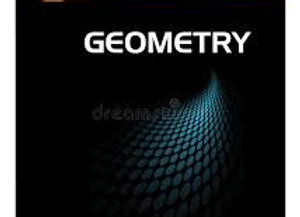# Geometry A / B

\$395.00Price
`In Geometry A, you will explore rigid and non-rigid transformations of figures in the coordinate plane and use them to establish congruence and similarity of triangles and other shapes. You will also prove theorems about lines, angles, triangles, and parallelograms, and build geometric constructions using both basic tools and modern technology. In conclusion, you will apply your knowledge of triangles as you investigate the mathematics of trigonometry.  In Geometry B, you will review the volume formulas for some common solid figures as you extend your knowledge of two-dimensional shapes to three-dimensional shapes. You will also transition from primarily Euclidean geometry to analytical geometry—a segment of geometry focused on numerical measurements and coordinate algebra. You will use analytical geometry and observations to investigate the properties of circles and constructions related to circles. Geometry B closes with a study of independent and conditional probability and how you can use probability models to represent situations arising in everyday life.`
Length